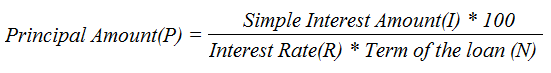Calculate Principal Amount using Interest Rate, Interest Amount, No. of Years | Simple Interest Calculator

# Calculate Principal Amount

## Calculate Principal Amount (P)

%

₹ 1,00,000
Principal Amount
₹ 60,000
Interest Amount
₹ 1,60,000
Total Amount
37.50%
Interest % to Total Amount

## Simple Interest Calculator

#### What is Simple Interest ?

Simple interest is a method to calculate the interest rate on a loan payment. Interest is always depends on the original principle or sum, so interest on interest is not included.

#### What is interest rate ?

Interest rate is the percentage at which interest accrued over time.

#### What is Interest Amount ?

Interest amount is the monthly simple interest payable

#### What is No. of Years ?

Time or period is the length of period in years.

## Formula to Calculate Principal Amount in Simple Interest

The principal amount in simple interest calculation can be calculated using given formulawhere

• I is the interest amount,
• P is the principal amount,
• R is the annual interest rate in % form, and
• N is the loan period expressed in years.